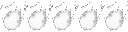### Souls Series Wiki Forums

Would you like to react to this message? Create an account in a few clicks or log in to continue.

2 posters

# Dark Souls Science: The rounding of level range formulasretro
ObsessedPosts : 441
Reputation : 127
Join date : 2012-05-26
One of the last confusing aspects of the level ranges was how the rounding worked, but I think we have a proper theory now. This should hopefully result in 100% accurate level range predictions.

---When you calculate the level range, the 10% part of the formula always has its decimal place truncated (or rounded down, depending on how you look at it). This occurs BEFORE completing the rest of the formula.

If 10% of your value is 2.5, what you really wind up with is actually just the number 2. So to find out the level range of a level 25 player using the white sign soapstone, it is:
25 +/- 12,
NOT
25 +/- 12.5.
If you do the latter, and try to round up or round down after the fact, both outcomes will actually be incorrect.

Here's another example. The Red Eye Orb invades only 10% below the invader's level (doesn't use the typical "10 +..." part of the formula). Without knowing how the rounding works correctly, a level 37 invader would probably assume that they can invade down to level 33 at the lowest.

This is because if you subtract 3.7 from 37, you get 33.3, which you'd expect to round down to 33. Or you might think that you're supposed to round 3.7 up to 4, also giving you an answer of 33. But that would be false. The decimal place needs to be truncated as soon as you calculate the 10% portion, so 3.7 turns into just 3. 37 - 3 = 34, so level 34 is actually the correct lowest level that could be invaded.Green__Eagle
CaffeinatedPosts : 712
Reputation : 51
Join date : 2012-01-18
Age : 62